#### Chapter 9 Triangle and its Angles R.D. Sharma Solutions for Class 9th MCQ's

Multiple Choice Questions

Mark the correct alternative in each of the following:

1. If all the three angles of a triangle are equal, then each one of them is equal to
(a) 90°
(b) 45°
(c) 60°
(d) 30°

Solution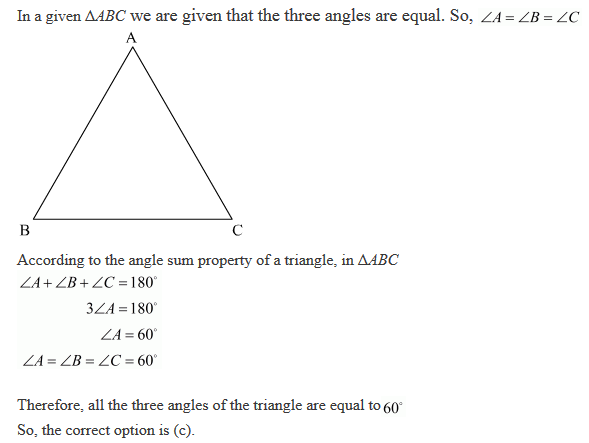2. If two acute angles of a right triangle are equal, then each acute is equal to
(a) 30°
(b) 45°
(c) 60°
(d) 90°

Solution3. An exterior angle of a triangle is equal to 100° and two interior opposite angles are equal. Each of these angles is equal to
(a) 75°
(b) 80°
(c) 40°
(d) 50°

Solution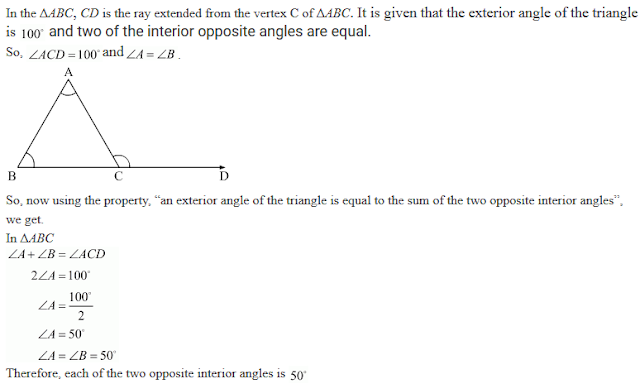4. If one angle of a triangle is equal to the sum of the other two angles, then the triangle is
(a) an isosceles triangle
(b) an obtuse triangle
(c) an equilateral triangle
(d) a right triangle

Solution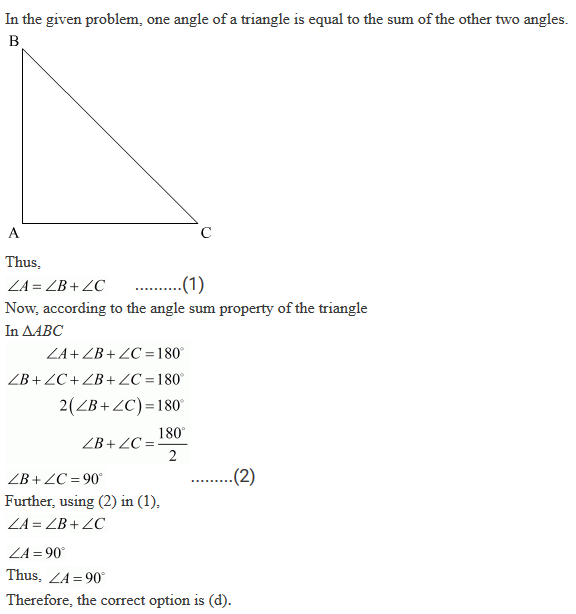5. Side BC of a triangle has been produced to a point D such that ∠ACD = 120°. If ∠B = 1/2 ∠A is equal to:
(a) 80°
(b) 75°
(c) 60°
(d) 90°

Solution6. In ΔABC, ∠B = ∠C and ray AX bisects the exterior angle ∠DAC. If ∠DAX = 70°, then ∠ACB =
(a) 35°
(b) 90°
(c) 70°
(d) 55°

Solution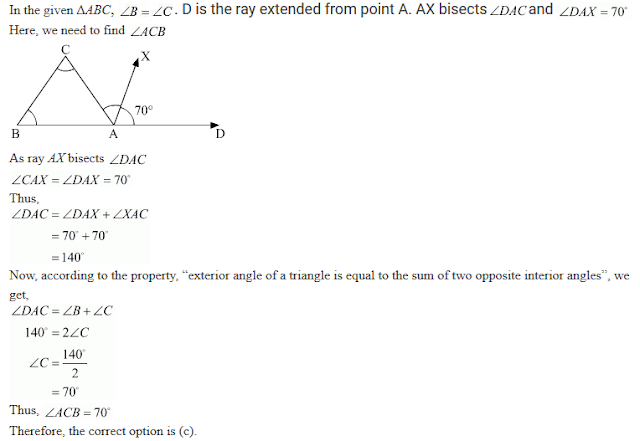7. In a triangle, an exterior angle at a vertex is 95° and its one of the interior opposite angle is 55°, then the measure of the other interior angle is
(a) 55°
(b) 85°
(c) 40°
(d) 9.0°

Solution8. If the sides of a triangle are produced in order, then the sum of the three exterior angles so formed is
(a) 90°
(b) 180°
(c) 270°
(d) 360°

Solution9. In ΔABC, if ∠A = 100°, AD bisects ∠A and AD ⊥ BC. Then, ∠B =

(a) 50°
(b) 90°
(c) 40°
(d) 100°

Solution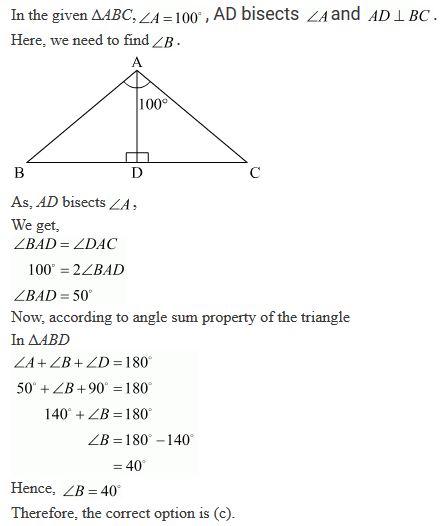10. An exterior angle of a triangle is 108° and its interior opposite angles are in the ratio 4 : 5. The angles of the triangle are
(a) 48°, 60°, 72°
(b) 50°, 60°, 70°
(c) 52°, 56°, 72°
(d) 42°, 60°, 76°

Solution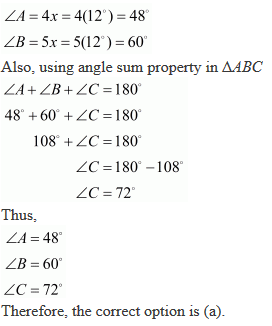11. In a ΔABC, if ∠A = 60°, ∠B = 80° and the bisectors of ∠B and ∠C meet at O, then ∠BOC =
(a) 60°
(b) 120°
(c) 150°
(d) 30°

Solution12. If the bisectors of the acute angles of a right triangle meet at O, then the angle at O between the two bisectors is
(a) 45°
(b) 95°
(c) 135°
(d) 90°

Solution13. Line segments AB and CD intersect at O such that AC || DB. If ∠CAB = 45° and ∠CDB = 55°, then ∠BOD =
(a) 100°
(b) 80°
(c) 90°
(d) 135°

Solution14.  The bisects of exterior angle at B and C of ΔABC meet at O. If ∠A = x°, then ∠BOC =
(a) 90° + x°/2
(b) 90° - x°/2
(c) 180° + x°/2
(d) 180° - x°/2

Solution15. In a ΔABC, ∠A =50° and BC is produced to a point D. If the bisectors of ∠ABC and ∠ACD meet at E, then ∠E=
(a) 25°
(b) 50°
(c) 100°
(d) 75°

Solution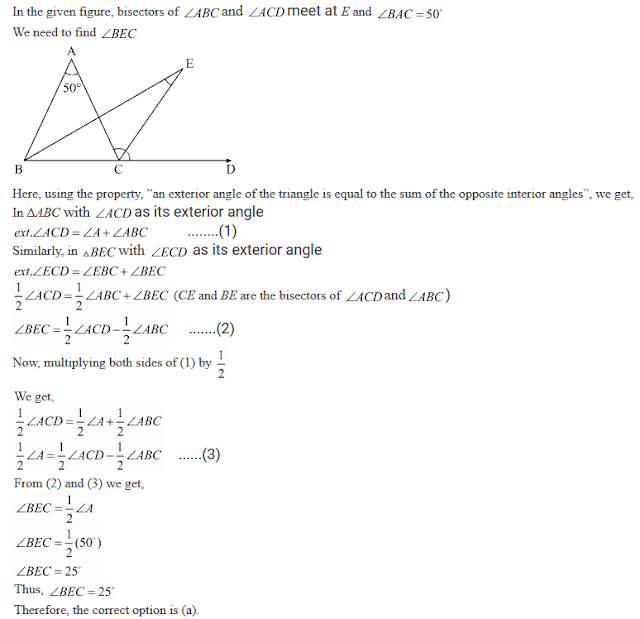16. The side BC of ΔABC is produced to a point D. The bisector of ∠A meets side BC in L. If ∠ACD = 115°, then ∠ALC=
(a) 85°
(b) (72 ½)°
(c) 145°
(d) none of these

Solution17. In Fig. 943, if EC∥AB, ∠ECD = 70° and ∠BDO = 20° and , then ∠OBD is
(a) 20°
(b)  20°
(c)  20°
(d) 70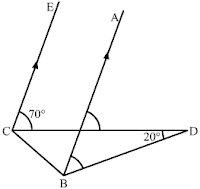Solution18. In Fig. 9.44, x + y =
(a) 270
(b) 230
(c) 210
(d) 190°Solution19. If the measures of angles of a triangle are in the ratio of 3 : 4 : 5, what is the measure of the smallest angle of the triangle?
(a) 25°
(b) 30°
(c) 45°
(d) 60°

Solution20. In Fig. 9.45 if AB⟂BC, then x =
(a) 18
(b) 22
(c) 25
(d) 32Solution21. In Fig 9.46, what is z in terms of x and y?

(a) x + y + 180
(b) x + y − 180
(c) 180° − (x + y)
(d) x + y + 360°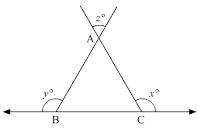Solution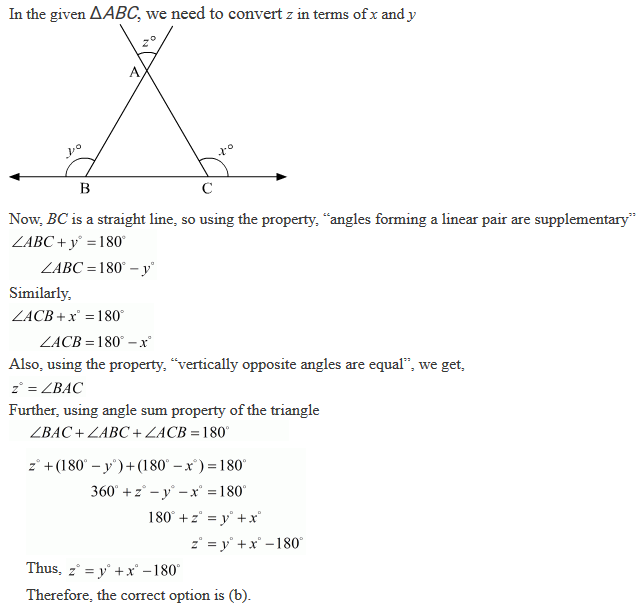22. In Fig. 9.47, for which the value of x is l1∥l2?
(a) 37
(b) 43
(c) 45
(d) 47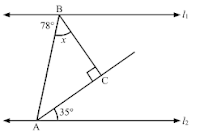Solution23. In Fig. 9.48, what is y in terms of x?
(a) 3/2 x
(b) 4/3 x
(c) x
(d) 3/4 x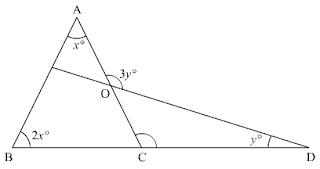Solution24. In Fig. .49, if l1∥l2, the value of x is:
(a) 22½
(b) 30
(c) 45
(d) 60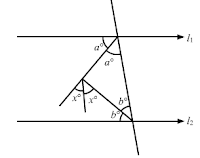Solution25 . In Fig. 9.50, what is the value of x?
(a) 35
(b) 45
(c) 50
(d) 60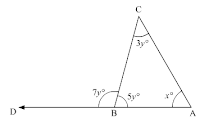Solution26. In △RST (See Fig. 9.51), what is the value of x?
(a) 40
(b) 0
(c) 80
(d) 100Solution27. In Fig. 9.52, the value of x is
(a) 65
(b) 80
(c) 95
(d) 120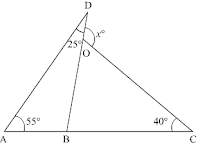Solution28. In Fig. 9.53, if BP∥CQ and AC=BC, then the measures of x is:
(a) 20
(b) 25
(c) 30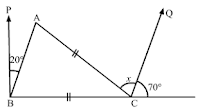Solution29. In Fig. 9.54, AB and CD are parallel lines and transversal EF intersects at P and Q respectively.
If ∠APR = 25, ∠RQC = 30 and ∠CQF = 65, then
(a) x = 55, y = 40
(b) x = 50, y = 45
(c) x = 60, y = 35
(d) x = 35, y = 60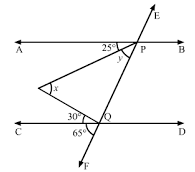Solution30. The base BC of triangle ABC is produced both ways and the measure of exterior angles formed are 94° and 126°. Then, ∠BAC =
(a) 94°
(b) 54°
(c) 40°
(d) 44°

Solution

In the given problem, the exterior angles obtained on producing the base of a triangle both ways are 94 and 126. So, let us draw ΔABC and extend the base BC, such that:
∠ACD = 126
∠ABE = 94Here, we need to find ∠BAC
Now, since BCD is a straight line, using the property, “angles forming a linear pair are supplementary”, we get
∠ACB + ∠ACD = 180
⇒ ∠ACB + 126 = 180
⇒ ∠ACB  = 180 - 126
⇒ ∠ACB = 54
Similarly, EBS is a straight line, so we get,
∠ABC + ∠ABE = 180
⇒ ∠ABC + 94 = 180
⇒ ∠ABC  = 180 - 94
⇒ ∠ABC = 86
Further, using angle sum property in ΔABC
∠ABC + ∠ACB + ∠BAC = 180
⇒ 54 + 86 + ∠BAC = 180
⇒ ∠BAC  = 180 - 140
⇒ ∠BAC = 40
Thus, ∠BAC = 40
Therefore, the correct option is (c).